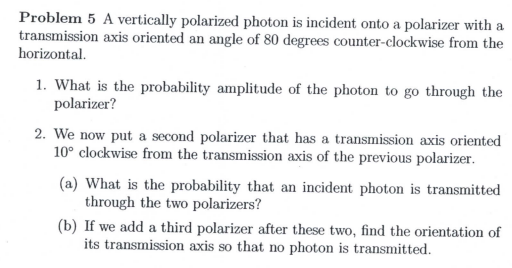# Problem 5 A vertically polarized photon is incident onto a polarizer with atransmission axis oriented an angle of 80 degrees counter-clockwise from thehorizontal1. What is the probability amplitude of the photon to go through thepolarizer?2. We now put a second polarizer that has a transmission axis oriented10° clockwise from the transmission axis of the previous polarizer(a) What is the probability that an incident photon is transmittedthrough the two polarizers?(b) If we add a third polarizer after these two, find the orientation ofits transmission axis so that nophoton is transmitted

Question
44 views

A note on this problem is "this beamsplitter IS polarization sensitive"help_outlineImage TranscriptioncloseProblem 5 A vertically polarized photon is incident onto a polarizer with a transmission axis oriented an angle of 80 degrees counter-clockwise from the horizontal 1. What is the probability amplitude of the photon to go through the polarizer? 2. We now put a second polarizer that has a transmission axis oriented 10° clockwise from the transmission axis of the previous polarizer (a) What is the probability that an incident photon is transmitted through the two polarizers? (b) If we add a third polarizer after these two, find the orientation of its transmission axis so that no photon is transmitted fullscreen
check_circle

Step 1

It is mentioned in the problem that the photon polarized in vertical direction is incident onto a polarizer.

Let the direction of polarization along the x axis be denoted by the ket |x> and the direction of polarization along the y direction be denoted as | y>

Let the angle which the transmission axis forms with the horizontal be denoted by θ.

Let the ket corresponding to the state of the transmitted photon when θ is measured counterclockwise from the horizontal x direction to the vertical y direction be denoted by | θ>

This ket | θ> can then be represented as a linear superposition of the linearly independent basis orthonormal basis kets   |x> and | y>  as,

Step 2

1)

The photon given in the problem is vertically polarized,

Therefore the probability amplitude for the photon to pass through the first polarizer is given by

Step 3

Since states |x> and | y>  are assumed to b...

### Want to see the full answer?

See Solution

#### Want to see this answer and more?

Solutions are written by subject experts who are available 24/7. Questions are typically answered within 1 hour.*

See Solution
*Response times may vary by subject and question.
Tagged in

### Physics